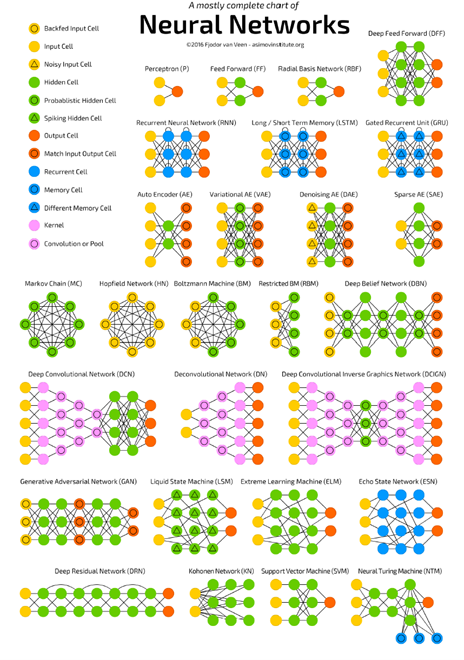# Neural Network based approaches for non-contact dermoscopy: a brief primer

Continuing our blog with introduction to machine learning for iToBoS, we present here a general overview of neural networks. Starting from basic definitions of important concepts, we will give a primer as meant for beginner. We keep discussions only to various types of neural networks. The functionality is also elucidated here.

In computer science, artificial neural networks (ANNs) are a form of machine learning modelled on biological neural networks. NNs are also known as "artificial neural systems" or "parallel distributed processing systems" or "relationship systems".

Artificial Neural Network (ANN) with more than two hidden layers is called as Deep Neural

Network (DNN). The smallest DNN had 3 hidden layers which was considered to be the earliest form of DNN. ANNs come in a variety of architectures such as Multilayer Perceptron (MLP), Convolutional Neural Network (CNN), and Long Short-Term Memory (LSTM). Every node has its own sequence of layers and connections. Among the many types of neural networks, the feedforward network is considered to be the simplest and most basic, as shown in figure 8. It consists of artificial neurons. It is a replica of the brain's neurons. During the action, the brain's neurons pass signals whereas in a neural network, artificial neurons perform tasks. Their connections are termed weights.

Activation function

This is a function that allows the network to pass the weighted sum through and after that, activate the node or deactivate it. When a node is deactivated, it will not transmit information to the subsequent nodes. Various activation functions exist, for example, sigmoid which is a very common activation function but often fails in complex networks due to "vanishing gradients" . Transfer function is another name for it . With or without an activation function, a neural network simply behaves as a linear regression model.

Much of common mathematic functions serve as the building blocks namely, Binary Step Function, Linear Activation Function, Non-Linear Activation Function, Sigmoid / Logistic Activation Function, Tangens hyperbole. For brevity of the blog, the detail discussion is not presented. Suffice to say the selection of the activation function is individual’s prerogative.

Feed forward

Essentially, this is the process of feeding a neural network with a series of inputs, using its weights, in order to obtain a product score. This is followed by supplying the weights with an activation function and comparing it to the actual output called ground.

A feed forward neural network is an artificial neural network in which nodes are not connected in a cycle, as opposed to a recurrent neural network, in which paths are cycled. With a feed-forward model, information is only processed in one direction, so data can pass through multiple hidden nodes, but it always moves forward. In particular, for machine learning applications, Feedforward Neural Networks' simplified architecture can be an advantage. For instance, one may set up a series of feed forward neural networks, but with a mild intermediary to moderate them. In order to handle and process larger tasks, this process relies on many neurons.

Backpropagation

Backpropagation was first coined by Bryson and Ho in 1969 and is an essential part in deep learning neural networks and it helps to reduce the error for better learning. Backpropagation learning takes place by first forwarding the input features through the network and see if the output matches to the label. It adjusts its weights if the output does not match with the label and repeats the cycle again, whereas if output matches then learning has completed. The weight adjustment is done by distributing the blame for error among the contributing weights which is a complex task because in fully connected network there are many weights connected to each input, therefore, each weight has impact on more than one input. The aim is to reduce the error as much as possible between the actual and learned output.

Asimov institute's Fjodor van Veen has compiled a complete cheat sheet on NN topologies as shown here.Figure 1 A comprehensive chart for neural networks (courtesy Asimov Institute)